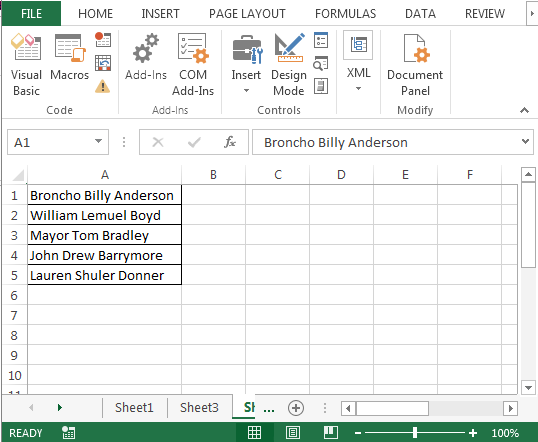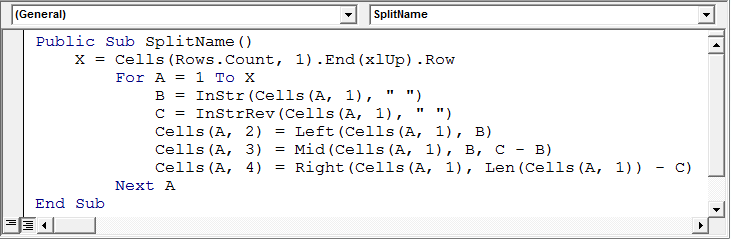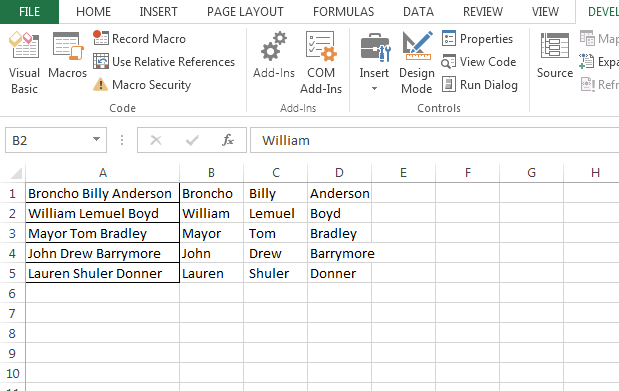How to Split Column through VBA

If you are facing trouble in splitting the text into two or more separate columns based on the specific character then you should read this article.

In this article, we are going to learn how to split the name into 3 cells, using VBA in Microsoft Excel.

Let’s take an example to understand where and how we can split the name.

We have a list of names in the format "FirstName Initial LastName" in column A. We want to split the entire column into three columns, with one containing all of the first names, second contains Initial and the third containing all of the last names.We will write the VBA code to split the name. Follow below given steps:-

To split the name into 3 cells, we will use VBA. See the below procedure and code, and you will understand it easily:-

• Open VBA Page and press Alt+F11
• Insert a module
• Then go to insert menu and click on procedure, where you will get the procedure to start the macro
• Write the below-mentioned code:-

Public Sub SplitName()
X = Cells(Rows.Count, 1).End(xlUp).Row
For A = 1 To X
B = InStr(Cells(A, 1), " ")
C = InStrRev(Cells(A, 1), " ")
Cells(A, 2) = Left(Cells(A, 1), B)
Cells(A, 3) = Mid(Cells(A, 1), B, C - B)
Cells(A, 4) = Right(Cells(A, 1), Len(Cells(A, 1)) - C)
Next A
End SubCode Explanation:- First we have given the macro name, then we used x to define the data range, and then we used A, B and C to define how values should be picked from the cells.

• Press the key F5 to run the Macro in Excel
• When you will run the macro, the names will split in three columnsConclusion: In this way, we can split the names into 3 columns, using VBA in Microsoft Excel.We would love to hear from you, do let us know how we can improve, complement or innovate our work and make it better for you. Write us at info@exceltip.com

1.